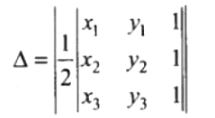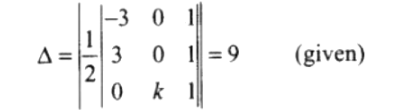# The area of a triangle with

Question:

The area of a triangle with vertices (–3, 0), (3, 0) and (0, k) is 9 sq. units. The value of will be

(A) 9

(B) 3

(C) – 9

(D) 6

Solution:

Option (B) 3

We know that, the area of a triangle with vertices (x1, y1), (x2, y2) and (x3, y3) is given byArea of triangle with vertices $(-3,0),(3,0)$ and $(0, k)$ is$[-3(-k)-0+1(3 k)]=\pm 18$

$6 k=\pm 18$

Thus,

$k=\pm \frac{18}{6}=\pm 3$